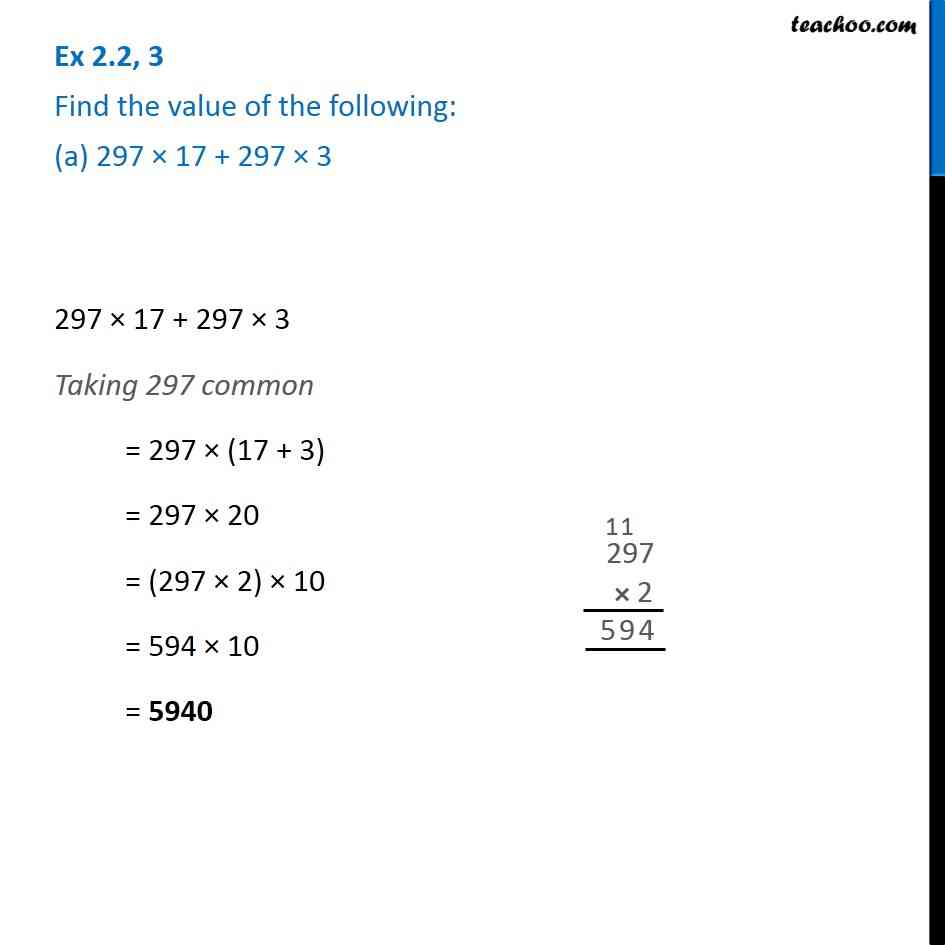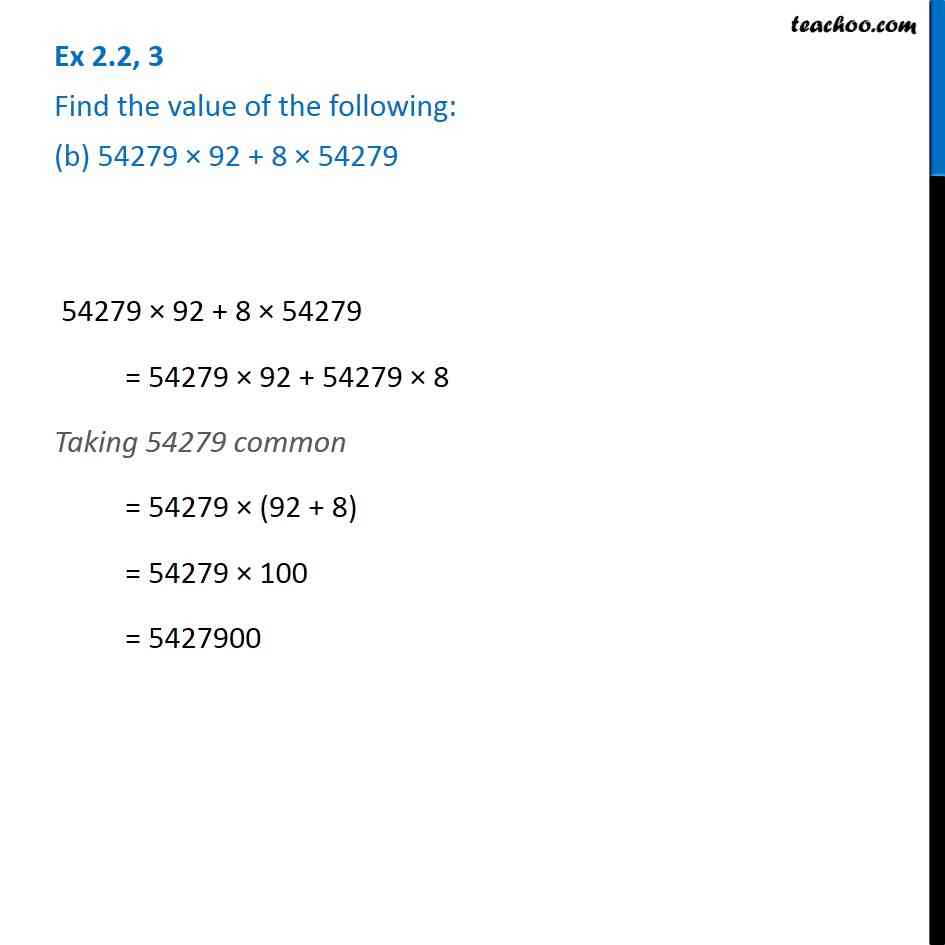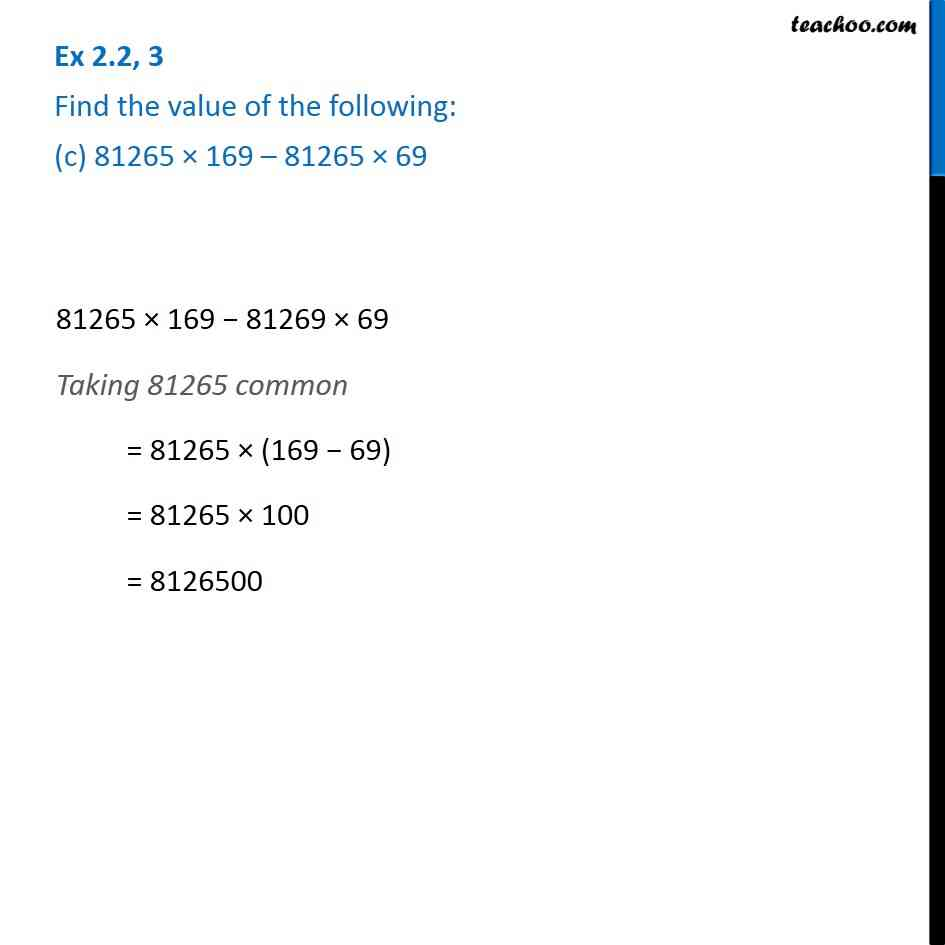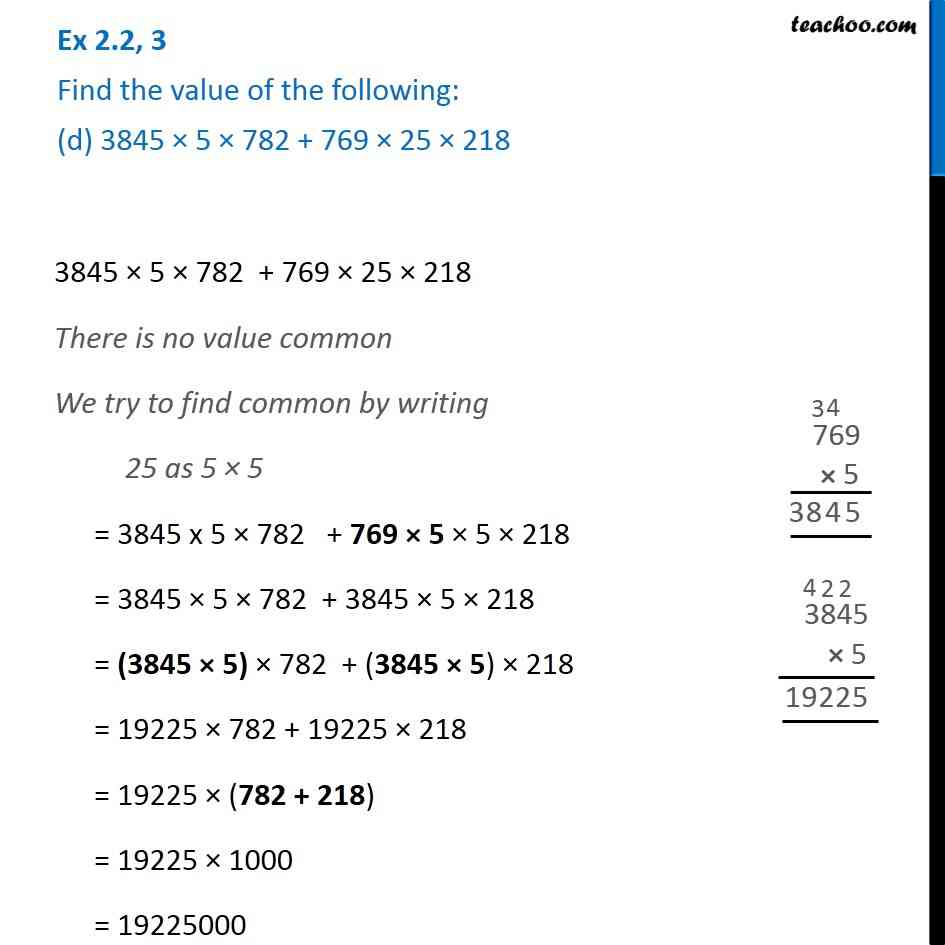Subscribe to our Youtube Channel - https://you.tube/teachoo

1. Chapter 2 Class 6 Whole Numbers
2. Serial order wise
3. Ex 2.2

Transcript

Ex 2.2, 3 Find the value of the following: (a) 297 × 17 + 297 × 3 297 × 17 + 297 × 3 Taking 297 common = 297 × (17 + 3) = 297 × 20 = (297 × 2) × 10 = 594 × 10 = 5940 Ex 2.2, 3 Find the value of the following: (b) 54279 × 92 + 8 × 54279 54279 × 92 + 8 × 54279 = 54279 × 92 + 54279 × 8 Taking 54279 common = 54279 × (92 + 8) = 54279 × 100 = 5427900 Ex 2.2, 3 Find the value of the following: (c) 81265 × 169 – 81265 × 69 81265 × 169 − 81269 × 69 Taking 81265 common = 81265 × (169 − 69) = 81265 × 100 = 8126500 Ex 2.2, 3 Find the value of the following: (d) 3845 × 5 × 782 + 769 × 25 × 218 3845 × 5 × 782 + 769 × 25 × 218 There is no value common We try to find common by writing 25 as 5 × 5 = 3845 x 5 × 782 + 769 × 5 × 5 × 218 = 3845 × 5 × 782 + 3845 × 5 × 218 = (3845 × 5) × 782 + (3845 × 5) × 218 = 19225 × 782 + 19225 × 218 = 19225 × (782 + 218) = 19225 × 1000 = 19225000

Ex 2.2